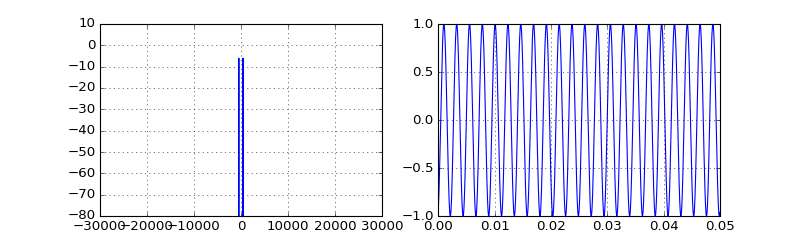# Hands-on Digital transmission with your soundcard¶

## 02 - Using the simulated audio channel¶

In the previous notebook, we set up the audio environment, listened to the transmitted signal from the loudspeaker and visualized the recorded signal from the microphone. However, since soon hearing the signal all the time could be annoying we used a cable to shortcut the connection between audio output and audio input.

Another possibility is to replace the actual audio transmission with a simulation of the propagation channel. Moreover, in contrast to the real audio channel, the behaviour of a simulated channel is reproducible, hence it can help debugging your signal processing chain. Therefore, in this notebook, we perform the same experiment as before, but use a simulated channel object.

So, first let's import the standard python packages:

In :
# Source code has been redacted in online version
# Omitting 10 lines of source code

In :
# Source code has been redacted in online version
# Omitting 4 lines of source code

In :
%matplotlib notebook


Subsequently, import the classes needed for the experiment:

In :
from audioComms import Environment, TX
from audioComms.plotting import PlotSpectrum, PlotWaveform


In addition, let's import the SimulatedChannel and a custom channel effect:

In :
from audioComms.channels import SimulatedChannel, IdentityChannelEffect


The channel effect describes the actual behaviour of the channel. For example, common soundcards have a frequency range of 20Hz-20kHz, so a reasonable channel effect is to apply a bandpass to the transmitted signal. However for simplicity, here we use an ideal channel, which does not change the transmitted signal at all.

Now, let's setup our transmitter again:

In :
class TransmitSine(TX):
def __init__(self, environment, Fc=440):
super().__init__(environment)
self._Fc = Fc            # the frequency of the sine wave
self._numsamples = 0     # the number of transmitted samples

def _generateSignal(self):
N = 10000
t = (np.arange(N) + self._numsamples) / self._samplerate
self._numsamples += N

return np.sin(2*np.pi*self._Fc*t)


Similar to the previous notebook, the data flow for the subsequent experiment looks as follows:

In :
%%tikz -l positioning
\tikzset{block/.style={draw,thick,minimum width=2cm,minimum height=1cm}}

\node (T) [block] {TransmitSine};
\node (C) [block,right=of T]  {SimulatedChannel};
\node (P1) [block,right=of C] {PlotSpectrum};
\node (P2) [block,below right=of C] {PlotWaveform};

\draw [-stealth] (T) -- (C);
\draw [-stealth] (C) -- (P1);
\draw [-stealth] ([xshift=0.5cm]C.east) |- (P2);Eventually, let's setup the experiment and run it:

In :
# 1 - Create the environment
samplerate = 44100
env = Environment(samplerate)

# 2 - Setup the components
tx = TransmitSine(environment=env, Fc=440)
channel = SimulatedChannel(environment=env, channelEffect=IdentityChannelEffect())
fig = env.figure(figsize=(10,3))
showSpec = PlotSpectrum(environment=env, windowDuration=1, logScale=True, axes=fig.add_subplot(121))

# 3 - Establish connections between the blocks
tx.transmitsTo(channel)
channel.transmitsTo(showSpec)
channel.transmitsTo(showWave)

# 4 - run the experiment
env.run()Stop received from external


As seen, the simulated channel also simply forwards the signal, which is then plotted as a spectrum and waveform.

### Summary and Outlook¶

With this notebook, we have finished setting up our programming environment. Let's get hands on real data transmission in the following notebook, where we will transmit a pulse-shaped signal in baseband and show the resulting eye diagram.